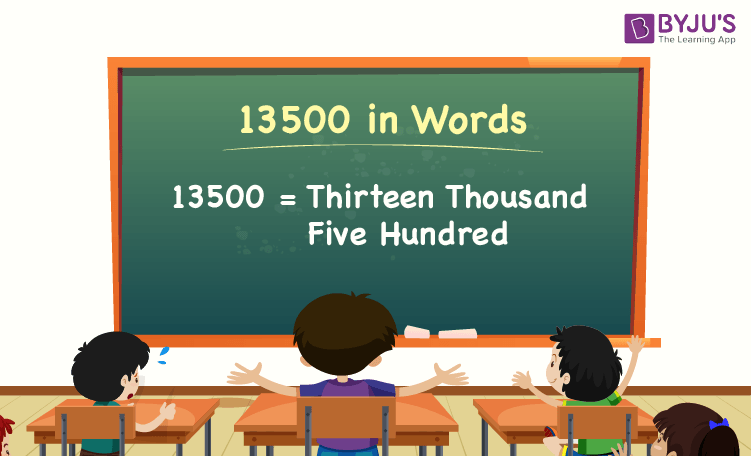# 13500 in Words

13500 in words is Thirteen Thousand Five Hundred. For example, if you earned Rs. 13500 in a month, you can write, “I have earned Rs. Thirteen thousand five hundred in a month”. In general, the number name of any number can be written using the ones, tens, hundreds, and thousands place of a number. Thus, the place value chart is useful for writing the number 13500 in words.

 13500 in Words: Thirteen Thousand Five Hundred

In this article, we will learn how to write 13500 in words, and look at the solved examples in detail.

## How to Write 13500 in Words?To write the number name of 13500, first, we have to determine the place value of each digit of the given number 13500. Hence, for the number 13500,

1’s place of 13500 is 0

10’s place of 13500 is 0.

100’s place of 13500 is 5.

1000’s place of 13500 is 13.

The following chart represents the place value of the number 13500:

 Thousands Hundreds Tens Ones 13 5 0 0

Therefore, the number 13500 in words is Thirteen Thousand Five Hundred.

### Examples

Example 1:

Find the value of 11000 + 2500. Describe the value in words.

Solution:

Given expression: 11000 + 2500

⇒ 11000 + 2500 = 13500

So, the value of 11000 + 2500 is 13500.

Hence, 13500 in words is thirteen thousand five hundred.

Example 2:

Express the value of thirteen thousand five hundred plus five thousand in words.

Solution:

Thirteen thousand five hundred = 13500

Five thousand = 5000

Thirteen thousand five hundred plus five thousand = 13500 + 5000 = 18500

Hence, the value of thirteen thousand five hundred plus five thousand is eighteen thousand five hundred in words.

Stay tuned to BYJU’S – The Learning App, and download the app to learn all Maths concepts quickly by exploring more learning videos.

## Frequently Asked Questions on 13500 in Words

Q1

### Write 13500 in words.

13500 in words can be written as thirteen thousand five hundred.

Q2

### Express the value 14000 – 500 in words.

Simplifying 14000 – 500, we get 13500. Hence, 13500 in words is written as thirteen thousand five hundred.

Q3

### How to write thirteen thousand five hundred in numbers?

Thirteen thousand five hundred in numbers is 13500.

Q4

### Is the number 13500 an odd number?

No, the number 13500 is not an odd number.

Q5

### Is 13500 a composite number?

Yes, 13500 is a composite number.

Test your Knowledge on 13500 in Words